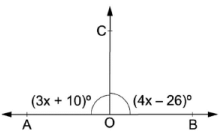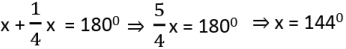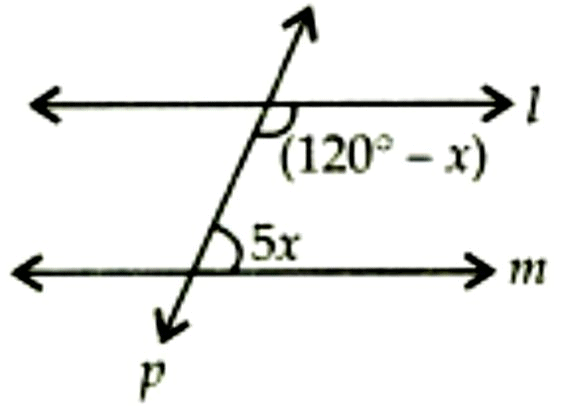GRE  >  Test: Lines & Angles- Assertion & Reason Type Questions

# Test: Lines & Angles- Assertion & Reason Type Questions - GRE

Test Description

## 10 Questions MCQ Test Quantitative Reasoning for GRE - Test: Lines & Angles- Assertion & Reason Type Questions

Test: Lines & Angles- Assertion & Reason Type Questions for GRE 2023 is part of Quantitative Reasoning for GRE preparation. The Test: Lines & Angles- Assertion & Reason Type Questions questions and answers have been prepared according to the GRE exam syllabus.The Test: Lines & Angles- Assertion & Reason Type Questions MCQs are made for GRE 2023 Exam. Find important definitions, questions, notes, meanings, examples, exercises, MCQs and online tests for Test: Lines & Angles- Assertion & Reason Type Questions below.
Solutions of Test: Lines & Angles- Assertion & Reason Type Questions questions in English are available as part of our Quantitative Reasoning for GRE for GRE & Test: Lines & Angles- Assertion & Reason Type Questions solutions in Hindi for Quantitative Reasoning for GRE course. Download more important topics, notes, lectures and mock test series for GRE Exam by signing up for free. Attempt Test: Lines & Angles- Assertion & Reason Type Questions | 10 questions in 20 minutes | Mock test for GRE preparation | Free important questions MCQ to study Quantitative Reasoning for GRE for GRE Exam | Download free PDF with solutions
 1 Crore+ students have signed up on EduRev. Have you?
Test: Lines & Angles- Assertion & Reason Type Questions - Question 1

### DIRECTION : In the following questions, a statement of assertion (A) is followed by astatement of reason (R). Mark the correct choice as:Assertion : If angles ‘a ’ and ‘b’ form a linear pair of angles and a = 400, then b = 1500.Reason : Sum of linear pair of angles is always 1800.

Detailed Solution for Test: Lines & Angles- Assertion & Reason Type Questions - Question 1
We know that the sum of linear pair of angles is always 1800.

So, Reason is correct.

Now, a + b = 400 + 1500 = 1900 ≠ 1800

Hence, Assertion is not correct

Correct option is (d) Assertion (A) is false but reason (R) is true.

Test: Lines & Angles- Assertion & Reason Type Questions - Question 2

### DIRECTION : In the following questions, a statement of assertion (A) is followed by astatement of reason (R). Mark the correct choice as:Assertion : If two interior angles on the same side of a transversal intersecting two parallel lines are in the ratio 5 : 4, then the greater of the two angles is 1000.Reason : If a transversal intersects two parallel lines, then the sum of the interior angles on the same side of the transversal is 1800.

Detailed Solution for Test: Lines & Angles- Assertion & Reason Type Questions - Question 2
We know If a transversal intersects two parallel lines, then the sum of the interior angles on the same side of the transversal is 1800 hat the solution of the line will satisfy the equation of the line.

So, Reason is correct.

Let the angles be 5x and 4x

Since, these two angles are co-interior angles. So, we have 5x + 4x = 1800

⇒ 9x = 1800 ⇒ x = 200

Hence, greater angle = 5x = 5 x 200 = 1000

So, Assertion is also correct

Correct option is (a) Both assertion (A) and reason (R) are true and reason (R) is the correct explanation of assertion (A).

Test: Lines & Angles- Assertion & Reason Type Questions - Question 3

### DIRECTION : In the following questions, a statement of assertion (A) is followed by astatement of reason (R). Mark the correct choice as:Assertion: In the given figure, AOB is a straight line. If ∠AOC = (3x + 10)° and ∠BOC (4x − 26)°, then ∠BOC = 860Reason: The sum of angles that are formed on a straight line is equal to 180°.

Detailed Solution for Test: Lines & Angles- Assertion & Reason Type Questions - Question 3
We know that the sum of angles that are formed on a straight line is equal to 180°.

So, Reason is correct.

We have : ∠AOC + ∠BOC = 180° [Since AOB is a straight line ]

⇒ 3x + 10 + 4x − 26 = 180°

⇒ 7x = 196°

⇒ x = 28°

∴ ∠BOC = [4 × 28 − 26]°

⇒ ∠BOC = 86°.

So, Assertion (A) is also true.

Correct option is (a) Both assertion (A) and reason (R) are true and reason (R) is the correct explanation of assertion (A).

Test: Lines & Angles- Assertion & Reason Type Questions - Question 4

DIRECTION : In the following questions, a statement of assertion (A) is followed by a

statement of reason (R). Mark the correct choice as:

Assertion : An angle is 140 more than its complementary angle, then angle is 520.

Reason : Two angles are said to be supplementary if their sum of measure of angles is 1800.

Detailed Solution for Test: Lines & Angles- Assertion & Reason Type Questions - Question 4

We know that two angles are said to be supplementary if their sum of measure of angles is 1800.

So, Reason is correct.

Let the angle be x . Complement of x = (900 - x)

Since, the difference is 140, we have x – (900 – x) = 140

⇒ 2x = 1040

⇒ x = 520

So, Assertion is also correct

But reason (R) is not the correct explanation of assertion (A).

Correct option is (b) Both assertion (A) and reason (R) are true and reason (R) is not the correct explanation of assertion (A).

Test: Lines & Angles- Assertion & Reason Type Questions - Question 5

DIRECTION : In the following questions, a statement of assertion (A) is followed by a

statement of reason (R). Mark the correct choice as:

Assertion: The angles of a triangle are in the ratio 2 : 3 : 4. The largest angle of the triangle is 800.

Reason: The sum of all the interior angles of a triangle is 1800.

Detailed Solution for Test: Lines & Angles- Assertion & Reason Type Questions - Question 5
We know that the sum of all the interior angles of a triangle is 1800.

So, Reason (R) is true.

Let the angles of a triangle be 2x, 3x and 4x then we have 2x + 3x + 4x = 1800.

⇒ 9x = 1800.

⇒ x = 200.

Hence, Largest angle = 4 x 200 = 800.

So, Assertion (A) is also true.

Also, Reason (R) is a correct explanation of Assertion (A).

Correct option is (a) Both assertion (A) and reason (R) are true and reason (R) is the correct explanation of assertion (A).

Test: Lines & Angles- Assertion & Reason Type Questions - Question 6

DIRECTION : In the following questions, a statement of assertion (A) is followed by a

statement of reason (R). Mark the correct choice as:

Assertion : Supplement of angle is one fourth of itself. The measure of the angle is 1440.

Reason : Two angles are said to be supplementary if their sum of measure of angles is 1800.

Detailed Solution for Test: Lines & Angles- Assertion & Reason Type Questions - Question 6
We know that two angles are said to be supplementary if their sum of measure of angles is 1800.

So, Reason is correct.

Let the angle be x . Supplement of x = (¼)x

So,So, Assertion is also correct.

Also, reason (R) is the correct explanation of assertion (A).

Correct option is (a) Both assertion (A) and reason (R) are true and reason (R) is the correct explanation of assertion (A).

Test: Lines & Angles- Assertion & Reason Type Questions - Question 7

DIRECTION : In the following questions, a statement of assertion (A) is followed by a

statement of reason (R). Mark the correct choice as:

Assertion : A triangle can have two obtuse angles.

Reason : The sum of all the interior angles of a triangle is 1800.

Detailed Solution for Test: Lines & Angles- Assertion & Reason Type Questions - Question 7
We know that the sum of all the interior angles of a triangle is 1800.

So, Reason (R) is true.

Since the Sum of two obtuse angles will be more than 1800.

So, Assertion (A) is false.

Correct option is (d) Assertion (A) is false but reason (R) is true.

Test: Lines & Angles- Assertion & Reason Type Questions - Question 8

DIRECTION : In the following questions, a statement of assertion (A) is followed by a

statement of reason (R). Mark the correct choice as:

Assertion: The value of x from the adjoining figure, if l || m is 150.

Reason: If two parallel lines are intersected by a transversal, then each pair of corresponding angles so formed is equal.Detailed Solution for Test: Lines & Angles- Assertion & Reason Type Questions - Question 8
We know that If two parallel lines are intersected by a transversal, then each pair of corresponding angles so formed is equal.

So, Reason is correct.

Also, we know that If a transversal intersects two parallel lines, then the sum of the interior angles on the same side of the transversal is 1800.

From figure we have, 1200 – x + 5x = 1800

⇒ 4x = 1800 – 1200

⇒ 4x = 600

⇒ x = 150

So, Assertion is also correct.

But reason (R) is not the correct explanation of assertion (A).

Correct option is (b) Both assertion (A) and reason (R) are true and reason (R) is not the correct explanation of assertion (A).

Test: Lines & Angles- Assertion & Reason Type Questions - Question 9

DIRECTION : In the following questions, a statement of assertion (A) is followed by a

statement of reason (R). Mark the correct choice as:

Assertion : Sum of the pair of angles 1200 and 600 is supplementary.

Reason : Two angles, the sum of whose measures is 1800, are called supplementary angles.

Detailed Solution for Test: Lines & Angles- Assertion & Reason Type Questions - Question 9
We know that two angles are said to be supplementary if their sum of measure of angles is 1800.

So, Reason is correct.

Now, 1200 + 600 = 1800 ⇒ Sum of the pair of angles 1200 and 600 is supplementary.

So, Assertion is also correct.

Also, reason (R) is the correct explanation of assertion (A).

Correct option is (a) Both assertion (A) and reason (R) are true and reason (R) is the correct explanation of assertion (A).

Test: Lines & Angles- Assertion & Reason Type Questions - Question 10

DIRECTION : In the following questions, a statement of assertion (A) is followed by a

statement of reason (R). Mark the correct choice as:

Assertion : If two internal opposite angles of a triangle are equal and external angle is given to be 1100, then each of the equal internal angle is 550.

Reason : A triangle with one of its angle 900, is called a right triangle.

Detailed Solution for Test: Lines & Angles- Assertion & Reason Type Questions - Question 10
For Assertion: We know that the exterior angle is equal to the sum of its interior opposite angles. So, x + x = 1100.

⇒ 2x = 1100

⇒ x = 550

So, Assertion is correct

Also, we know that a triangle with one of its angle 900, is called a right triangle.

So, Reason is also correct.

But reason (R) is not the correct explanation of assertion (A).

Correct option is (b) Both assertion (A) and reason (R) are true and reason (R) is not the correct explanation of assertion (A).

## Quantitative Reasoning for GRE

90 videos|87 docs|130 tests
Information about Test: Lines & Angles- Assertion & Reason Type Questions Page
In this test you can find the Exam questions for Test: Lines & Angles- Assertion & Reason Type Questions solved & explained in the simplest way possible. Besides giving Questions and answers for Test: Lines & Angles- Assertion & Reason Type Questions, EduRev gives you an ample number of Online tests for practice

## Quantitative Reasoning for GRE

90 videos|87 docs|130 tests